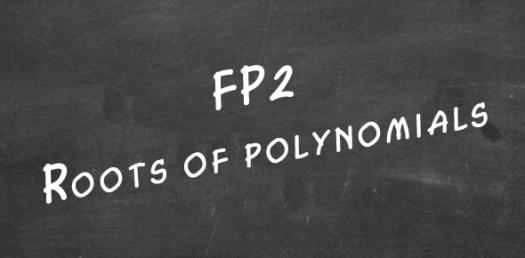# Roots Of Polynomial Equations

10 Questions | Attempts: 94
ShareSettingsMultiple choice questions.

• 1.
• A.

4

• B.

12

• C.
• D.

-4

• 2.
• A.
• B.

4

• C.

0

• D.

-4

• 3.
• A.

2-3i and 1

• B.

2-3i and 2

• C.

2+i and 2

• D.

3+2i and 1

• 4.
• A.
• B.
• C.
• D.
• 5.
• A.

-9 - 4i

• B.

2 - 3i

• C.

-2 + 3i

• D.

5 + 2i

• 6.
• A.

-12

• B.

12

• C.

24

• D.

-24

• 7.
• A.
• B.
• C.
• D.
• 8.

• A.
• B.
• C.
• D.
• 9.
• A.

P = -5 and q = -47

• B.

P = 5 and q = -47

• C.

P = 5 and q =47

• D.

P = -5 and q =47

• 10.
• A.

-1 , -3 and 4

• B.
• C.

1, 3 and -4

• D.

## Related TopicsBack to top
×

Wait!
Here's an interesting quiz for you.